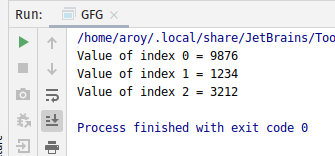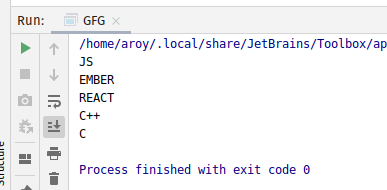# AtomicReferenceArray setPlain() method in Java with Examples

• Last Updated : 03 Jan, 2020

The setPlain() method of a AtomicReferenceArray class is used to set the value of the element at index i to newValue. Both index i and newValue are passed as parameters to the method. This method set the value with memory semantics of setting as if the variable was declared non-volatile and non-final.

Syntax:

```public final void setPlain(int i, E newValue)
```

Parameters: This method accepts:

• i which is an index of AtomicReferenceArray to perform the operation,
• newValue which is the new value to set.

Return value: This method returns nothing.

Below programs illustrate the setPlain() method:
Program 1:

 `// Java program to demonstrate``// setPlain() method`` ` `import` `java.util.concurrent.atomic.*;`` ` `public` `class` `GFG {`` ` `    ``public` `static` `void` `main(String[] args)``    ``{`` ` `        ``// create an atomic reference object.``        ``AtomicReferenceArray ref``            ``= ``new` `AtomicReferenceArray(``5``);`` ` `        ``// set some value and print``        ``ref.setPlain(``0``, ``9876``);``        ``ref.setPlain(``1``, ``1234``);``        ``ref.setPlain(``2``, ``3212``);`` ` `        ``System.out.println(``"Value of index 0 = "``                           ``+ ref.get(``0``));``        ``System.out.println(``"Value of index 1 = "``                           ``+ ref.get(``1``));``        ``System.out.println(``"Value of index 2 = "``                           ``+ ref.get(``2``));``    ``}``}`

Output:Program 2:

 `// Java program to demonstrate``// setPlain() method`` ` `import` `java.util.concurrent.atomic.*;`` ` `public` `class` `GFG {`` ` `    ``public` `static` `void` `main(String[] args)``    ``{`` ` `        ``// create an atomic reference object``        ``AtomicReferenceArray ref``            ``= ``new` `AtomicReferenceArray(``5``);`` ` `        ``// set some value``        ``ref.setPlain(``0``, ``"JS"``);``        ``ref.setPlain(``1``, ``"EMBER"``);``        ``ref.setPlain(``2``, ``"REACT"``);``        ``ref.setPlain(``3``, ``"C++"``);``        ``ref.setPlain(``4``, ``"C"``);`` ` `        ``// print``        ``for` `(``int` `i = ``0``; i < ``5``; i++) {``            ``System.out.println(ref.get(i));``        ``}``    ``}``}`

Output:My Personal Notes arrow_drop_up